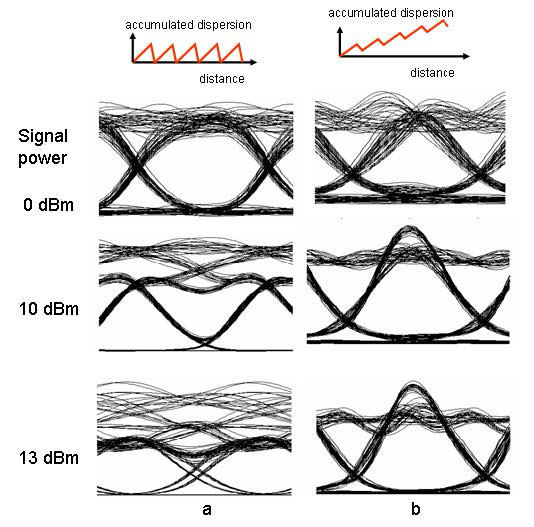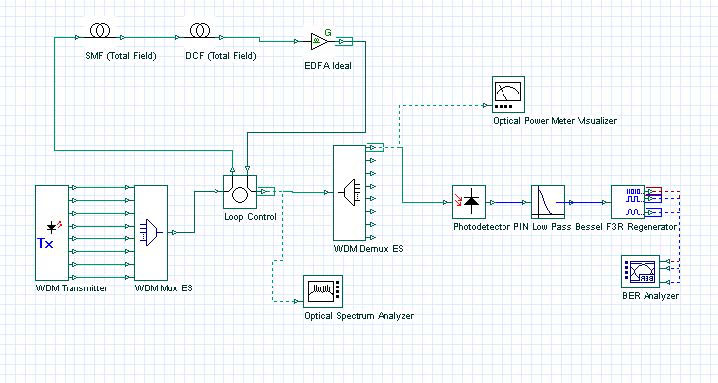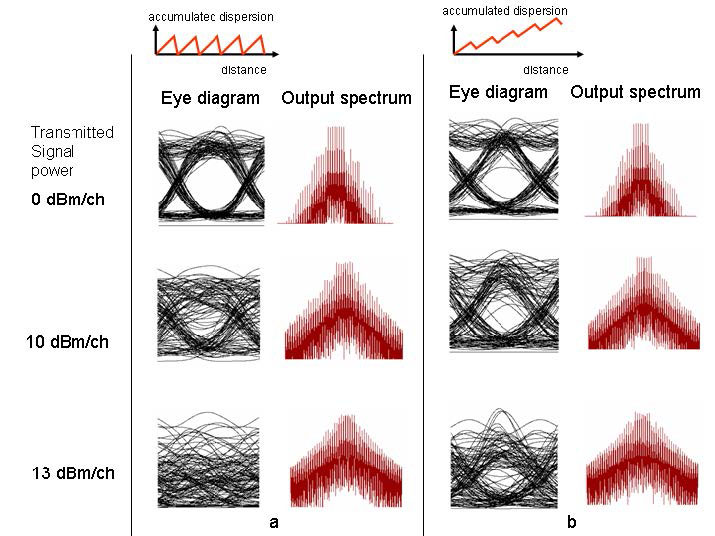The purpose of this example is to investigate the fiber nonlinearity and dispersion related issues in a system.

As long as the optical power within an optical fiber is small, the fiber can be treated as linear medium. However, when the power level is high, we have to consider the impact of nonlinear effects. Nonlinear effects can be classified into two categories:

• Refractive index related
• Scattering related

Some nonlinear effects occur in multi channel WDM systems where interaction of signals at different wavelengths is possible. See Table 1 for more information.

Table 1: Nonlinear effects in the fiber

 Category Single channel Multi-channel Refractive index related Self-phase modulation (SPM) Cross-phase modulation (XPM) Four wave mixing (FWM) Scattering related Stimulated Brillouin scattering (SBS) Stimulated Raman scattering (SRS)

SPM and XPM affect the phase of signals and cause spectral broadening, which in turn leads to increases in dispersion penalties. SBS and SRS provide gains to some channels by depleting power from other channels. The nonlinear interaction depends on transmission length and effective area of the fiber. Since loss in the fiber decreases the signal power, we use an effective instead of physical length. SPM can be a significant consideration in designing 10 Gbps systems, and it restricts the maximum channel power to below a few dBm. XPM becomes an important consideration when the channel spacing is tens of GHz. FWM efficiency depends on signal power and dispersion, as well as channel separation in WDM systems. If the channel is close to the zero dispersion wavelength of the fiber, considerably high power can be transferred to FWM components. Using unequal channel spacing can also reduce effect of FWM.

Dispersion plays a key role in reducing the effects of nonlinearities. However, dispersion itself can cause intersymbol interference. Fortunately, we can engineer systems with zero total dispersion but a certain amount of local dispersion along the link.

In the following example, we will consider the effects of dispersion compensation on system performance in a high power regime where nonlinearities are active. Initially, we will consider a dispersion post-compensated system with a single channel. The project is found in the Fiber nonlinearities and dispersion single channel.osd file. In this file, we have two different versions of the same project. In the first version, system residual dispersion is 0, whereas it is 800 ps/nm in the second version. The transmission link contains 5 spans and the bit rate is 10 Gbps. The dispersion of 100 km SMF is 16 ps/nm-km and its effective area is 72 square microns. The dispersion of DCF is -80 ps/nm-km. A 20 km DCF is used for the first version to totally compensate the dispersion. For the second version, an 18 km DCF is used to leave some residual dispersion after each span. This adds 800 ps/nm total residual dispersion to the system. The effective area of DCF is 30 square microns. SMF and DCF losses are compensated by an EDFA with 25 dB gain for the first version and with 24.4 dB gain for the second version. The Noise figure of the EDFA is 4 dB. If we consider only the SMF fiber, and include the effect of amplification (5 times), we can estimate the threshold power for SPM  . If we do so, we find that after about 10 dBm average power, SPM becomes a limiting effect. We need to investigate if this is the case, and how the residual system dispersion effects it. Figure 1 shows the eye diagrams for two different residual dispersion values and three different signal powers.Figure 1: Eye diagrams of the received signal for several received signal powers when system residual dispersion is a) 0, b) 800 ps/nm.

Note: Inset on the top shows the dispersion map for each case.

This simulation shows that effect of SPM can be reduced by not completely compensating the dispersion and leaving some residual dispersion in the system. Notice how power increase results closes the eye in the case of zero residual dispersion.

## Multi-channel system

Let us now consider a multi-channel system with 8 channels. For this example, the project layout is shown in Figure 2. The first channel is at 193.1 THz and the channels are separated by 100 GHz. SMF and DCF parameters are the same as in the previous example. In this example, we set the sample per bit parameter to, so as to eliminate effect in fibers. For illustrative purposes, we have set the maximum nonlinear phase shift parameter of the fibers to 100 mrad. This is done to get a fast result. To get more accurate results, this parameter should be set to a lower value. Simulation results are shown in Figure 3.Figure 2: Project layoutFigure 3: Eye diagrams of the received signal for several signal powers when a) fiber dispersion is 0; b) and c) when system residual dispersion is 0 and 800 ps/nm respectively.

Note: Insets show the power spectrums and dispersion maps for each case.

For an eight-channel system, the threshold power is approximately 10 dBm per channel . In this simulation, both SPM and XPM affect the system performance. The simulation also shows that nonlinear effects can be reduced by local dispersion and better performance is obtained with nonzero residual dispersion .

References

          G. P. Agrawal, Fiber Optic Communication Systems, Wiley-Interscience, 1997.

          R. Ramaswami and K. N. Sivarajan, Optical Networks: A practical Perspective, Morgan Kaufmann, 1998.

          G. Bellotti et. al., “Dependence of self-phase modulation impairments on residual dispersion in 10 Gb/s based terrestrial transmission using standard fiber”, IEEE Photon. Tech. Lett. 11, pp.  824, 1999.